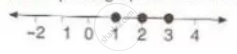Share

# Find the Values of X Which Satisfy the Inequation -2 <= 1/2 - (2x)/3 < 1 5/6; X ∈ N Graph the Solution on the Number Line - Mathematics

Course

#### Question

Find the values of x which satisfy the inequation

-2 <= 1/2 - (2x)/3 < 1 5/6; x ∈ N

Graph the solution on the number line

#### Solution

-2 <= 1/2 - (2x)/3 < 1 5/6

-2 <= 1/2 - (2x)/3   and 1/2 - (2x)/3 < 1 5/6

(-5)/2 <= - (2x)/3  and -(2x)/3 < 8/6

15/4 >= x and x > -2

3.75 >= x and x > -2

since x ∈ N

∴ Solution set = {1, 2, 3}

The required graph of the solution set is:Is there an error in this question or solution?